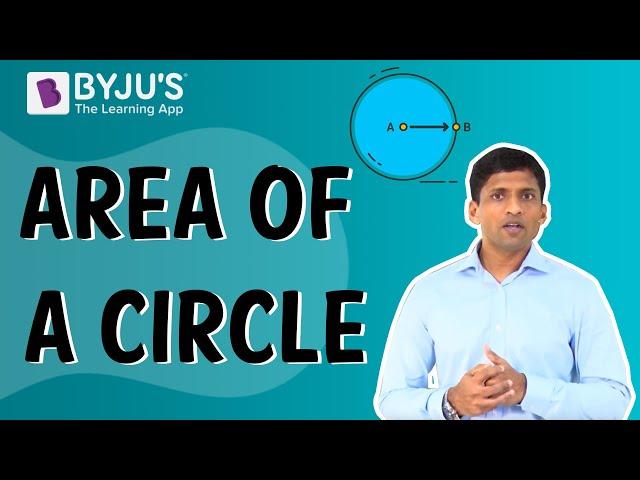# Conic Sections Class 11 Notes - Chapter 11Let us consider a fixed vertical line (l) and let (m) be another line intersecting line (l) at any fixed point V. Let α be the angle of intersection between line l and m. If line m is rotated around line l such that the angle of intersection (α) remains constant. Then, the resultant surface is a double-napped circular hollow cone. Where V is known as the vertex, l is the axis and m is known as the generator of the resultant cone. The vertex separating the cone into 2 different parts is known as the nappes. The section obtained by the intersection of a cone with the plane is known a the conic section. Based on the angle and position of the intersecting plane w.r.t. cone, different types of conic sections can be obtained.

### Conditions for Ellipse, Circle, Hyperbola and Parabola

When a plane cuts (other than vertex) the nappe of the cone, we have following conditions:

• When
$$\begin{array}{l}\beta = 90^{0}\end{array}$$
, the resultant is a circle
• When
$$\begin{array}{l}\alpha < \beta < 90^{0}\end{array}$$
, the resultant is an ellipse
• When
$$\begin{array}{l}\beta = \alpha\end{array}$$
, the resultant is a parabola
• When
$$\begin{array}{l}0 \leq \beta < \alpha\end{array}$$
, the resultant is a hyperbola

### Degenerated Sections

When a plane cuts the vertex of the cone, we get following cases:

• The section is a point when,
$$\begin{array}{l}\alpha < \beta \leq 90^{0}\end{array}$$
• Parabola degeneration: When
$$\begin{array}{l}\beta = \alpha\end{array}$$
, the resultant section is a straight line.
• Hyperbola degeneration: When
$$\begin{array}{l}0 \leq \beta < \alpha\end{array}$$
, the resultant section is the pair of intersecting straight lines.

A set of points equidistant from a fixed point (center) in a plane is known the circle. Let ‘r’ be the radius of the circle and O(h, k) be the coordinate of its center. Let P(a, b) be any point on the circle. Then, the equation of the circle is given by:

$$\begin{array}{l}\sqrt{\left ( a-h \right )^{2}+\left ( b-k \right )^{2}=r}\end{array}$$
[distance formula]

If the equation of parabola has y2 then, the symmetrical axis is along the x-axis and if the equation of parabola has x2, then the symmetrical axis is along the y-axis.

When the symmetrical axis is along the x-axis the parabola opens to the

• Right, if x has a +ve coefficient,
• Left, if x has -ve coefficient.

When the symmetrical axis is along the y-axis the parabola opens

• Upwards, if y has a +ve coefficient
• Downwards, if y has -ve coefficient.

### Conic Sections Class 11 Practice Questions

1. Find the equations of the hyperbola if,
• Vertices (± 5, 0), foci (± 6, 0)
• Vertices (0, ± 7), foci (0, ± 3)
• Vertices (0, ± 4), foci (0, ± 5)
• Foci (± 8, 0), length of transverse axis: 12.
• Foci (0, ±13), length of conjugate axis: 23.
• Foci (± 5 , 0), length of latus rectum: 44.
• Foci (± 14, 0), length of latus rectum: 45.
1. Find the equation for the ellipse if,
• Vertices (± 8, 0), foci (± 2, 0)
• Vertices (0, ± 23), foci (0, ± 15)
• Vertices (± 16, 0), foci (± 9, 0)
1. Find the equation of the circle if,
• centre (0,12) and radius 42,
• centre (9, -13) and radius 8

## Frequently asked Questions on CBSE Class 11 Maths Notes Chapter 11: Conic Sections

### What is a ‘Conic Section’?

In geometry, any curve produced by the intersection of a plane and a right circular cone.